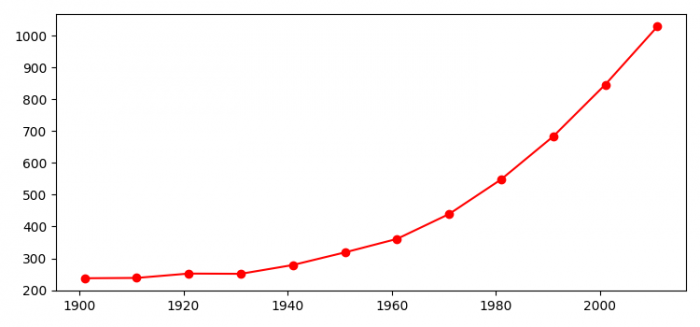# How to create a line chart using Matplotlib?

To create a line chart using matplotlib, we can take the following steps −

• Set the figure size and adjust the padding between and around the subplots.
• Make lists of years and population growth.
• Plot years and population on the line using plot() method.
• To display the figure, use show() method.

## Example

from matplotlib import pyplot as plt

plt.rcParams["figure.figsize"] = [7.50, 3.50]
plt.rcParams["figure.autolayout"] = True

years = [1901, 1911, 1921, 1931, 1941, 1951,
1961, 1971, 1981, 1991, 2001, 2011]
population = [237.4, 238.4, 252.09, 251.31, 278.98,
318.66, 361.09, 439.23, 548.16,
683.33, 846.42, 1028.74]

plt.plot(years, population, color='red', marker='o')

plt.show()

## OutputUpdated on: 04-Aug-2021

621 Views